• 拓扑排序 在有向图 中经常用来判断 是否存在 环. 若一个有向图中 存在 拓扑排序,则 一定不存在 环 若存在 环 ,则一定不存在 拓扑排序 举个例子 : 假设有 n 个变量,有 m 个二元组 (u,v),分别表示 变量u 小于 v.那么,...
拓扑排序 在有向图 中经常用来判断 是否存在 环. 若一个有向图中 存在 拓扑排序,则 一定不存在 环 若存在 环 ,则一定不存在 拓扑排序

举个例子 : 假设有 n 个变量,有 m 个二元组 (u,v),分别表示 变量u 小于 v.那么,所有变量从小到大排列起来.

例如:四个变量a,b,c,d,若已知 a < b,c < b ,d < c,则这 4 个变量的排序 可能是 a < d < c < b (答案不唯一,为什么?)
如图: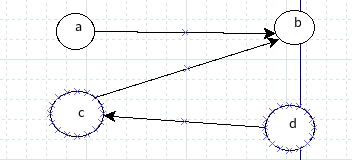每次遍历 找到 当前入度为 0 的点,然后删除该点以及以该点为起点的边.
首先删掉结点 a ,及 a->b 的边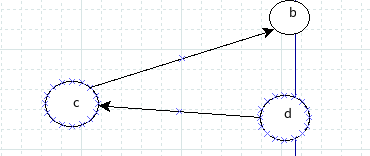然后 删掉 d 及 d->c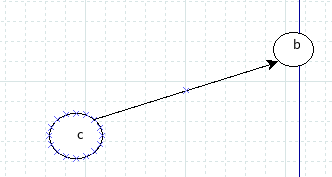删掉 c 及 c->b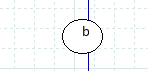删除结点的顺序 就为 拓扑排序后的顺序 (a -> d -> c -> b)
因为 开始时,a 和 d的 入度都为 0,所以答案不唯一 . 也可能是 d -> a -> c -> b
裸 拓扑排序

思路: 统计 各个结点的 入度 ,然后 维护一个 队列, 当 存在一个结点 的入度为 0, 将改点加入队.然后进入循环,若不存在 入度为0的点,则不存在拓扑排序.然后 以 队列 中元素为起点的边,将 其 终点的入度 减一 ,判断改点的入度是否为 0.若为0,加入队列.不为 0,则继续循环,当队列中没有元素时,退出循环. 记录进入队列元素的个数 cnt. 若 cnt == 总的点个数.则不存在环,进入队列的顺序就为拓扑排序的后的顺序.

核心代码
int toposort()
{
while(!q.empty())  q.pop();
for(int i = 0;i < n;i++)   if(!indeg[i]) q.push(i);

while(!q.empty())
{
int temp = q.front();
cnt++;
q.pop();

for(int i = 0;i < v[temp].size();i++)
{
int x = v[temp][i];
indeg[x]--;
if(!indeg[x]) q.push(x);
}
}

if(cnt == n)   return 1;
else return 0;

}

(1)练习拓扑排序 --(板子题)
和(1)很类似稍微加了点需求
核心代码
virus[x] 表示第 x 个结点 的 病毒 数 (注意取余)
   virus[x] = (virus[x] + virus[temp])%mod;

最后进行求和 最后注意取余
               sum += virus[i];
sum = sum%mod;

展开全文算法
• ## 拓扑排序入门（真的很简单）

万次阅读 多人点赞 2018-06-25 19:15:30
在一个有向图中，对所有的节点...如果最后不存在入度为0的节点，那就说明有环，不存在拓扑排序，也就是很多题目的无解的情况。 下面是算法的演示过程。 下面是我以前的写法，比较好理解，但是效率低 //b[]...
在一个有向图中，对所有的节点进行排序，要求没有一个节点指向它前面的节点。
先统计所有节点的入度，对于入度为0的节点就可以分离出来，然后把这个节点指向的节点的入度减一。
一直做改操作，直到所有的节点都被分离出来。
如果最后不存在入度为0的节点，那就说明有环，不存在拓扑排序，也就是很多题目的无解的情况。
下面是算法的演示过程。下面是我以前的写法，比较好理解，但是效率低
 //b[]为每个点的入度
for(i=1;i<=n;i++){
for(j=1;j<=n;j++){
if(b[j]==0){   //找到一个入度为0的点
ans=j;
vis[cnt++]=j;
b[j]--;
break;
}
}
for(j=1;j<=n;j++)
if(a[ans][j]) b[j]--; //与入度为0的点相连的点的入度减一
}
printf("%d",vis);
for(i=1;i<cnt;i++) printf(" %d",vis[i]);
printf("\n");


下面是我现在一直以来的写法，O(V+E)。点数+边书

    queue<int>q;
vector<int>edge[n];
for(int i=0;i<n;i++)  //n  节点的总数
if(in[i]==0) q.push(i);  //将入度为0的点入队列
vector<int>ans;   //ans 为拓扑序列
while(!q.empty())
{
int p=q.front(); q.pop(); // 选一个入度为0的点，出队列
ans.push_back(p);
for(int i=0;i<edge[p].size();i++)
{
int y=edge[p][i];
in[y]--;
if(in[y]==0)
q.push(y);
}
}
if(ans.size()==n)
{
for(int i=0;i<ans.size();i++)
printf( "%d ",ans[i] );
printf("\n");
}
else printf("No Answer!\n");   //  ans 中的长度与n不相等，就说明无拓扑序列
有些拓扑排序要求字典序最小什么的，那就把队列换成优先队列就好了。
例如：ZCMU-2153点击打开链接
代码：
#include<bits/stdc++.h>
using namespace std;
typedef long long LL;
const int inf=1e9;
const int maxn=1e6+5;
vector<int>edge;
int in;
int main()
{
char s;
set<int>k;
while(cin>>s)
{
k.insert(s-'A');
k.insert(s-'A');
if(s=='>')
{
in[s-'A']++;
edge[s-'A'].push_back(s-'A');
}
else
{
in[s-'A']++;
edge[s-'A'].push_back(s-'A');
}
}
priority_queue<int,vector<int>,greater<int> >q;
for(int i=0;i<30;i++)
{
if(in[i]==0&&k.count(i)!=0)
q.push(i);
}
vector<int>ans;
while(!q.empty())
{
int p=q.top(); q.pop();
ans.push_back(p);
for(int i=0;i<edge[p].size();i++)
{
int y=edge[p][i];
in[y]--;
if(in[y]==0&&k.count(y)!=0)
q.push(y);
}
}
if(ans.size()==k.size())
{
for(int i=0;i<ans.size();i++)
printf("%c",ans[i]+'A');
printf("\n");
}
return 0;
}
还有一种比较坑的排序 要求编号小的尽量排在前面，这里与字典序最小是不一样的，看一下例题。
HDU-4857 点击打开链接

逃生

Time Limit: 2000/1000 MS (Java/Others)    Memory Limit: 32768/32768 K (Java/Others) Total Submission(s): 6725    Accepted Submission(s): 1965

Problem Description
糟糕的事情发生啦，现在大家都忙着逃命。但是逃命的通道很窄，大家只能排成一行。 现在有n个人，从1标号到n。同时有一些奇怪的约束条件，每个都形如：a必须在b之前。 同时，社会是不平等的，这些人有的穷有的富。1号最富，2号第二富，以此类推。有钱人就贿赂负责人，所以他们有一些好处。 负责人现在可以安排大家排队的顺序，由于收了好处，所以他要让1号尽量靠前，如果此时还有多种情况，就再让2号尽量靠前，如果还有多种情况，就让3号尽量靠前，以此类推。 那么你就要安排大家的顺序。我们保证一定有解。

Input
第一行一个整数T(1 <= T <= 5),表示测试数据的个数。 然后对于每个测试数据，第一行有两个整数n(1 <= n <= 30000)和m(1 <= m <= 100000)，分别表示人数和约束的个数。 然后m行，每行两个整数a和b，表示有一个约束a号必须在b号之前。a和b必然不同。

Output
对每个测试数据，输出一行排队的顺序，用空格隔开。

Sample Input
 
15 103 51 42 51 23 41 42 31 53 51 2

Sample Output
 
1 2 3 4 5

举个例子如图：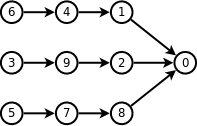如果你用优先队列拓扑排序得到的是：3 5 6 4 1 7 8 9 2 0
但是正确答案为 6 4 1 3 9 2 5 7 8 0 这样使得小的（1）尽量在前面。
这里我们可以得到 前面的小的不一定排在前面，但是有一点后面大的一定排在后面。
我们看 6和3不一定3排在前面，因为6后面连了一个更小的数字1能使得6更往前排。
在看 2和 8，8一定排在后面，因为8后面已经没有东西能使它更往前排（除了0）。
所以最后我们的做法就是 建立一个反图，跑一边字典序最大的拓扑排序，最后再把这个排序倒过来就是答案了。
#include<iostream>
#include<cstdio>
#include<cstring>
#include<algorithm>
#include<vector>
#include<queue>
using namespace std;
typedef long long ll;
vector<int>edge,ans;
priority_queue<int>q;
int in;
int T,n,m;
void init()
{
for(int i=1;i<=n;i++)
{
edge[i].clear();
in[i]=0;
}
while(!q.empty()) q.pop();
ans.clear();
}
void solve()
{
int i,j;
for(i=1;i<=n;i++)
if(in[i]==0) q.push(i);
while(!q.empty())
{
int p=q.top(); q.pop();
ans.push_back(p);
for( i=0; i<edge[p].size(); i++ )
{
int v=edge[p][i];
in[v]--;
if(in[v]==0) q.push(v);
}
}
for(i=ans.size()-1;i>0;i--)
printf("%d ",ans[i]);
printf("%d\n",ans);
}
int main()
{
int a,b;
scanf("%d",&T);
while(T--)
{
scanf("%d%d",&n,&m);
init();
while(m--)
{
scanf("%d%d",&a,&b);
edge[b].push_back(a);
in[a]++;
}
solve();
}
return 0;
}



展开全文• ## 拓扑排序

千次阅读 2017-02-28 00:01:59
拓扑排序
一个无环的有向图称为无环图（Directed Acyclic Graph），简称DAG图。
在一个表示工程的有向图中，用顶点表示活动，用弧表示活动之间的优先关系，这样的有向图为顶点表示活动的网，我们称之为AOV网（Active On Vertex Network）。
拓扑序列：设G=(V,E)是一个具有n个顶点的有向图，V中的顶点序列V1,V2,……,Vn满足若从顶点Vi到Vj有一条路径，则在顶点序列中顶点Vi必在顶点Vj之前。则我们称这样的顶点序列为一个拓扑序列。
现在举个例子，如下图所示: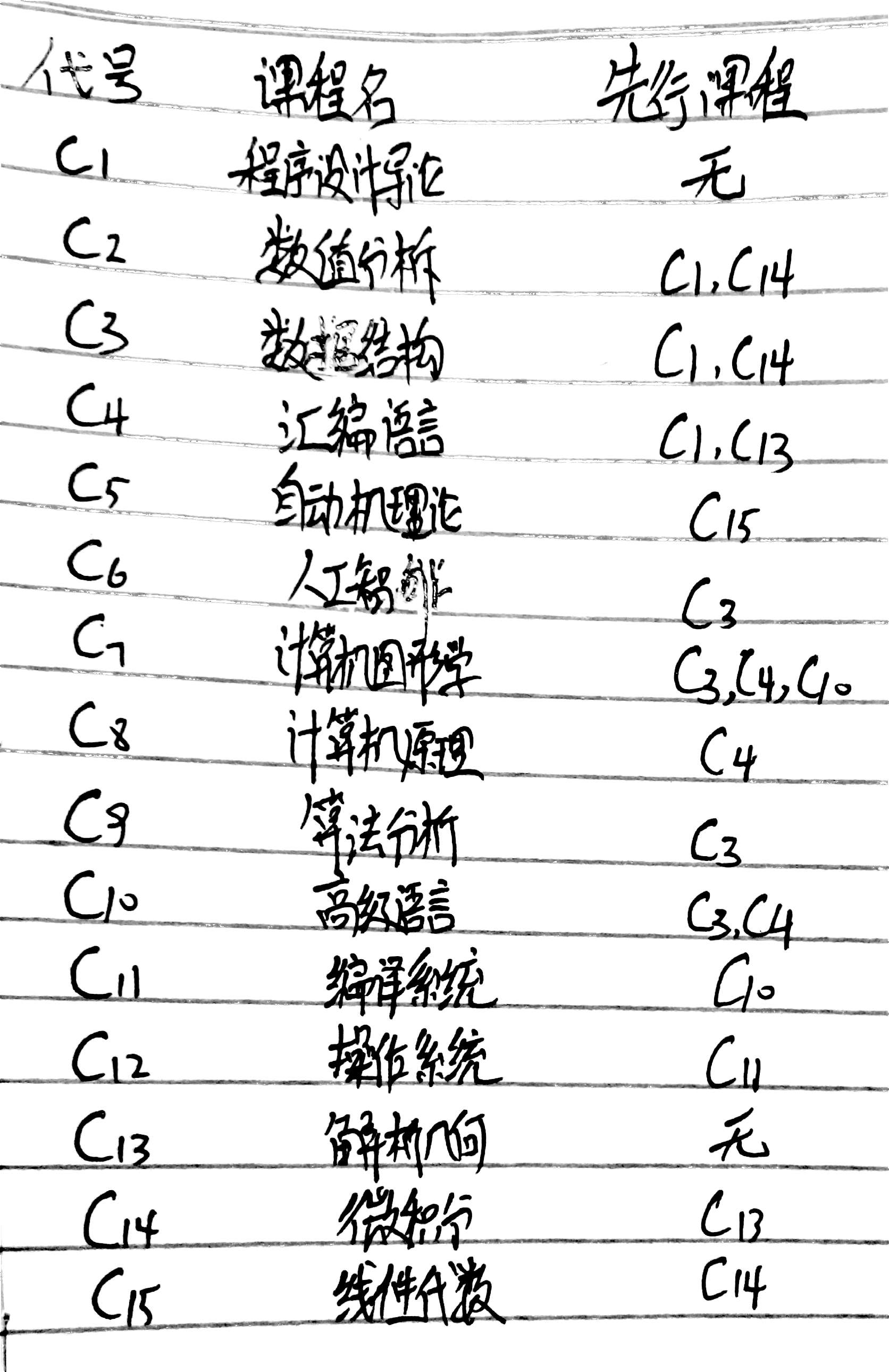其中拓扑图如下：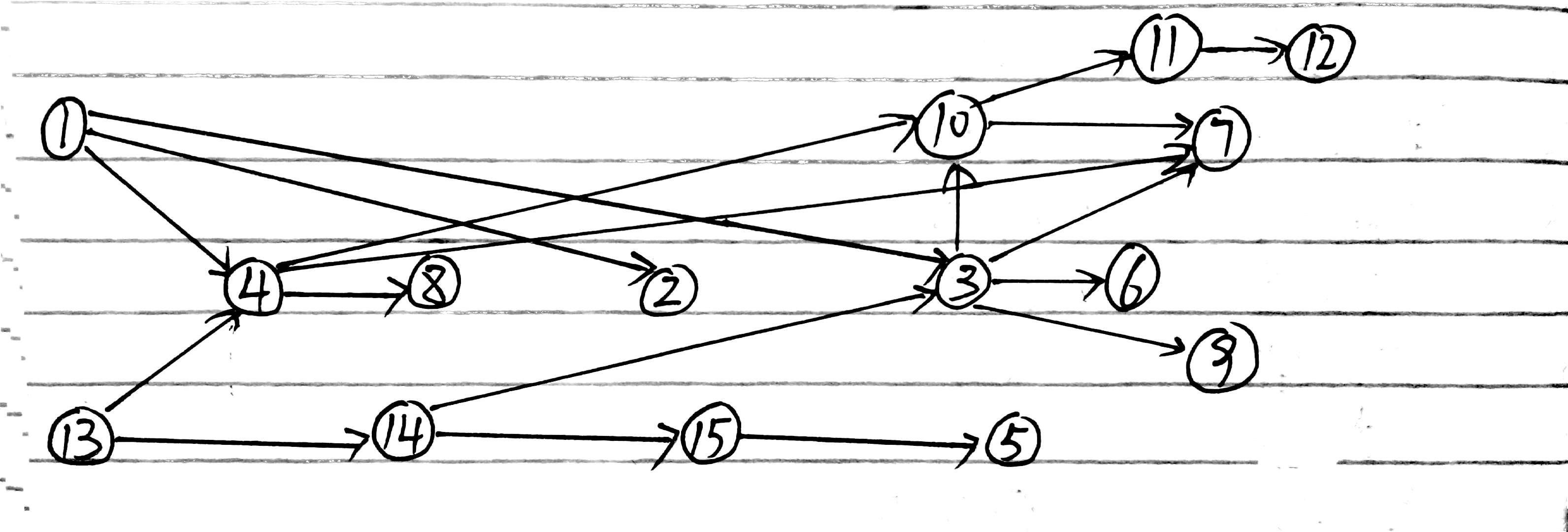可以做邻接矩阵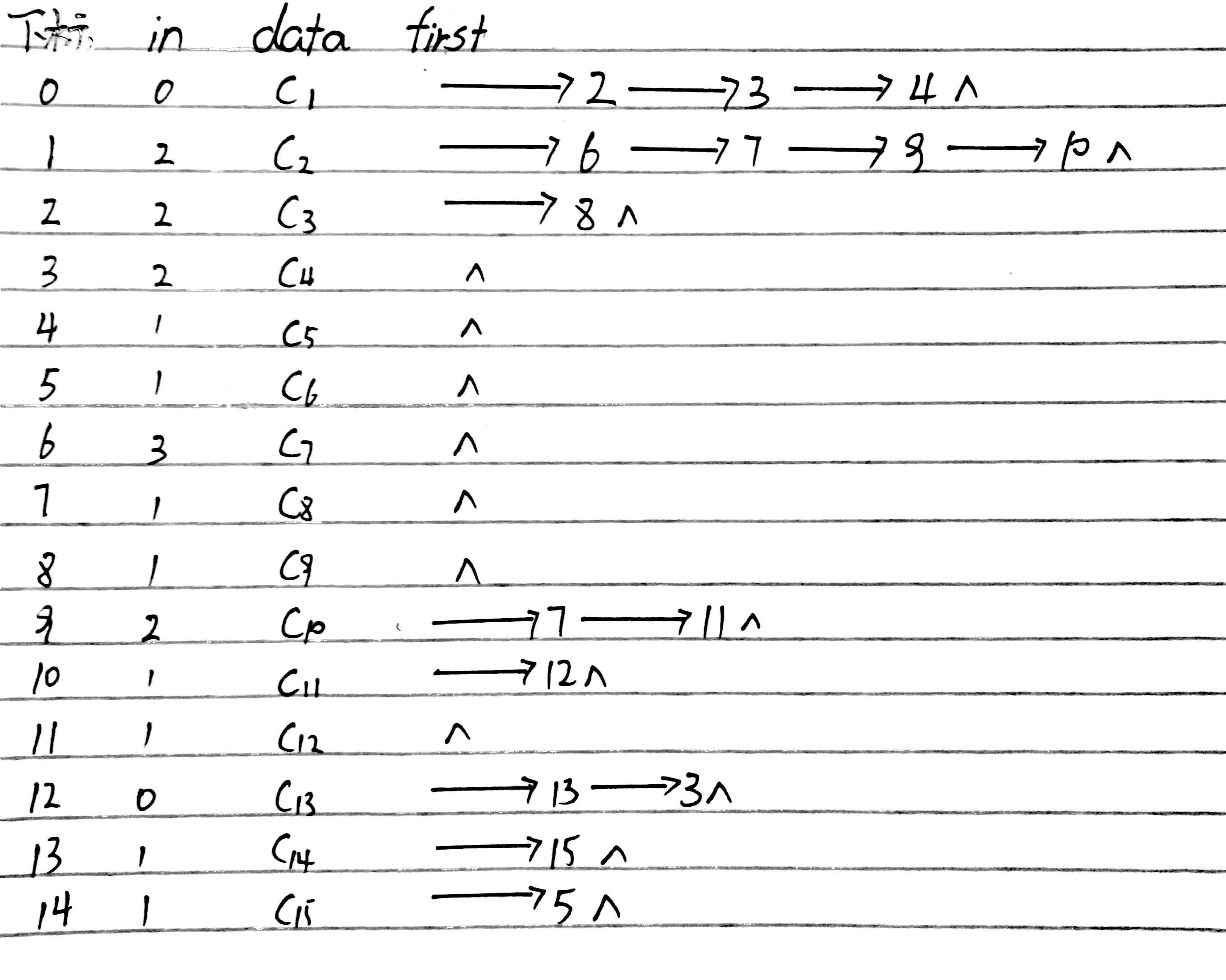下面是代码：

// 边表结点声明
typedef struct EdgeNode
{
struct EdgeNode *next;
}EdgeNode;

// 顶点表结点声明
typedef struct VertexNode
{
int in;			// 顶点入度
int data;
EdgeNode *firstedge;

typedef struct
{
int numVertexes, numEdges;

// 拓扑排序算法
// 若GL无回路，则输出拓扑排序序列并返回OK，否则返回ERROR
{
EdgeNode *e;
int i, k, gettop;
int top = 0;		// 用于栈指针下标索引
int count = 0;		// 用于统计输出顶点的个数
int *stack;			// 用于存储入度为0的顶点

stack = (int *)malloc(GL->numVertexes * sizeof(int));

for( i=0; i < GL->numVertexes; i++ )
{
{
stack[++top] = i;	// 将度为0的顶点下标入栈
}
}

while( 0 != top )
{
gettop = stack[top--];	// 出栈
count++;

{
// 注意：下边这个if条件是分析整个程序的要点！
// 将k号顶点邻接点的入度-1，因为他的前驱已经消除
// 接着判断-1后入度是否为0，如果为0则也入栈
{
stack[++top] = k;
}
}
}

if( count < GL->numVertexes )	// 如果count小于顶点数，说明存在环
{
return ERROR;
}
else
{
return OK;
}
}


展开全文数据结构 结构 c语言 排序...